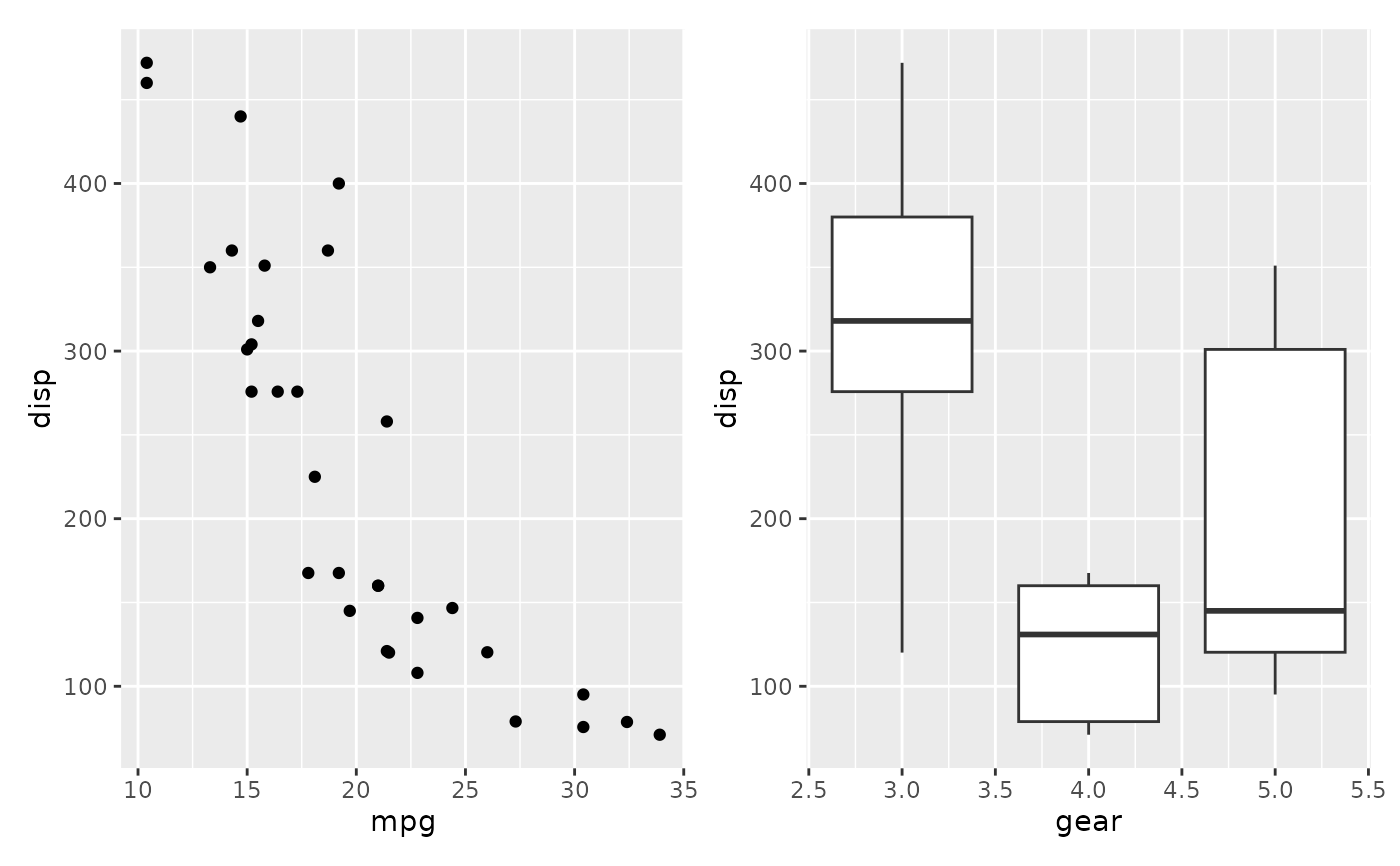The 'ggplot2' package provides a strong API for sequentially building up a plot, but does not concern itself with composition of multiple plots. 'patchwork' is a package that expands the API to allow for arbitrarily complex composition of plots by, among others, providing mathematical operators for combining multiple plots. Other packages that try to address this need (but with a different approach) are 'gridExtra' and 'cowplot'.

## Overview

The use and premise of patchwork is simple: Just add ggplot2 plots together to compose multiplot layouts. Because of this simplicity there is not much more to say. Still, a few functions allow you to modify the behaviour, e.g.:

• plot_layout() allows you to define the grid that plots are put into

• plot_annotation() allows you to add titles, tags etc.

The guides below will teach you all about what you can do with patchwork.

## Author

Maintainer: Thomas Lin Pedersen thomasp85@gmail.com (ORCID)

## Examples

library(ggplot2)
# You can add plots saved to variables

p1 <- ggplot(mtcars) + geom_point(aes(mpg, disp))
p2 <- ggplot(mtcars) + geom_boxplot(aes(gear, disp, group = gear))

p1 + p2# Or build it up in one step
ggplot(mtcars) +
geom_point(aes(mpg, disp)) +
ggplot(mtcars) +
geom_boxplot(aes(gear, disp, group = gear))